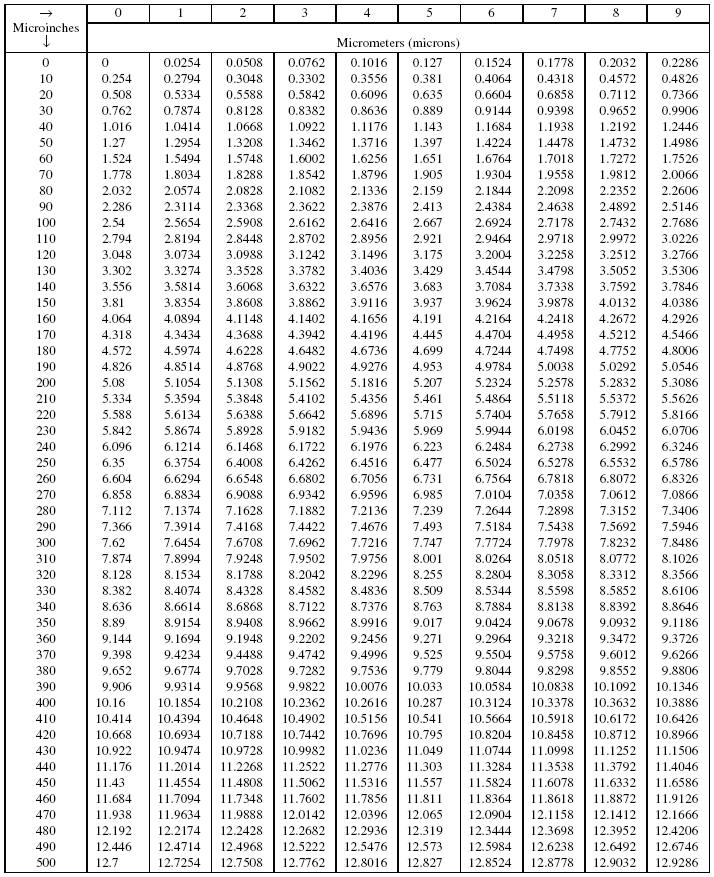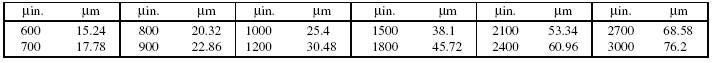Microinches to Micrometers (microns) ConversionUse the small table below to convert microinches to micrometers for ranges higher than given in the main table above. Appropriate quantities chosen from both tables are simply added to obtain the higher converted value:Both tables based on 1 microinch = 0.0254 micrometers, exactly. All values in both parts of this
table are exact; figures to the right of the last place figures are all zeros.

Example: Convert 1375 μin. to μm:

From lower portion of Table: 1200 μin. = 30.48 μm
From upper portion of Table: 175 μin.  = 4.445 μm
1375 μin. = 34.925 μm

Recherche personnalisée